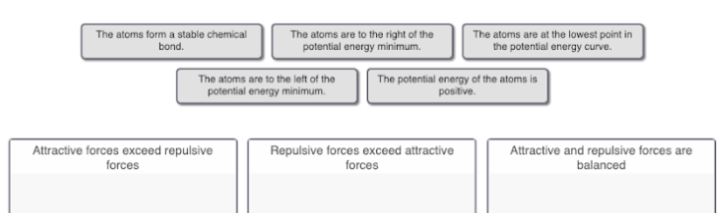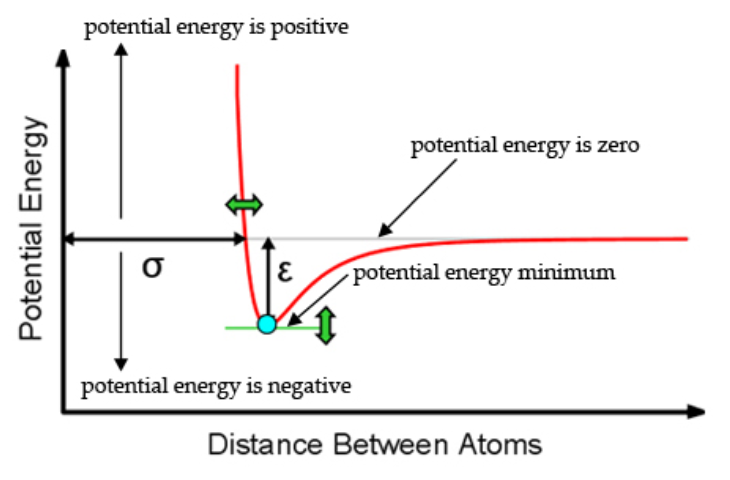# Problem: Part A. According to your observations, classify the following statements based on the forces present between the atoms.Drag the appropriate items to their respective bins.Valence bond theory explains that a bond is formed when two atomic orbitals overlap.The graph that compares the distance between atoms (x axis) versus the potential energy of the atoms (y axis) is known as the potential energy curve. This potential energy curve shows how the potential energy of the system changes as the two atoms come together to form a chemical bond This curve is unique for every set of atoms that form a bond.In the potential energy curve created for you in the simulation, consider the gray line parallel to the x axis to be a potential energy of zero. The potential energy is negative below the gray line and positive above the gray line. The potential energy minimum (lowest point) indicates where a chemical bond is formed. The bond length is the distance between the two atoms when the potential energy is lowest in the potential energy curve.

###### FREE Expert Solution
86% (312 ratings)View Complete Written Solution
###### Problem Details

Part A. According to your observations, classify the following statements based on the forces present between the atoms.Drag the appropriate items to their respective bins.

Valence bond theory explains that a bond is formed when two atomic orbitals overlap.

The graph that compares the distance between atoms (x axis) versus the potential energy of the atoms (y axis) is known as the potential energy curve. This potential energy curve shows how the potential energy of the system changes as the two atoms come together to form a chemical bond This curve is unique for every set of atoms that form a bond.

In the potential energy curve created for you in the simulation, consider the gray line parallel to the x axis to be a potential energy of zero. The potential energy is negative below the gray line and positive above the gray line. The potential energy minimum (lowest point) indicates where a chemical bond is formed. The bond length is the distance between the two atoms when the potential energy is lowest in the potential energy curve.